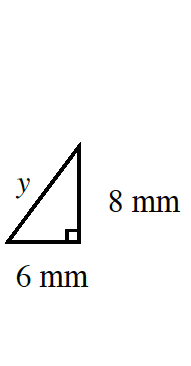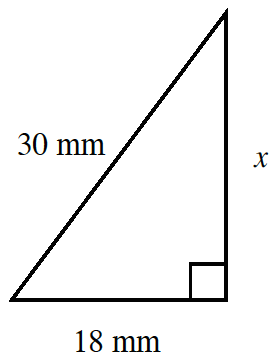### Home > CC3 > Chapter Ch6 > Lesson 6.2.5 > Problem6-92

6-92.

Sketch the two similar triangles at right on your own paper. Find the scale factor and the missing side lengths.$\cdot\ ? \ \Rightarrow$Since the scale factor applies to all sides of the shape equally, the two triangles share a common side ratio.

Based on the triangles above, use the given side lengths to find the scale factor ratio between the two triangles.
While each triangle is missing a certain side length, do you notice a pair of sides that are both apparent?

Use the scale factor you have solved for to determine the missing side lengths by either multiplying or dividing.

Scale Factor: $3:1$
Now try solving for $y$ and $x$.